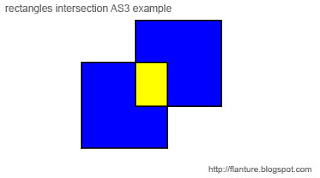ActionScript3.0 Rectangle class is part of the flash.geom package besides Point, Matrix, Transform and ColorTransform. Any visual representation of rectangles has to be implemented by programmer. One way of doing it is to create new class and here I wanted to include rectangle color as initial parameter, so this new class vRectangle (from visual rectangle, off course) looks something like this:

vRectangle.as
`package{import flash.geom.Rectangle;import flash.display.Shape;import flash.display.Sprite;public class vRectangle{public var rect:Rectangle;public var shape:Shape;public function vRectangle(a:Number, b:Number, c:Number, d:Number, e:uint):void{this.rect = new Rectangle(a, b, c, d);this.shape = new Shape();this.shape.graphics.beginFill(e);this.shape.graphics.lineStyle(2, 0x000000);this.shape.graphics.drawRect(rect.x, rect.y, rect.width, rect.height);this.shape.graphics.endFill();}}}`
Class has 4 parameters to define a - rectangle's x position, b - rectangle's y position, c - rectangle's width and d - rectangle's height one additional parameter e - which defines rectangle's color.

This is important because some Rectangle Class methods like intersection and union return Rectangle as result and I wanted to make distinction between objects by using different color.First example creates two rectangles using newly created vRectangle class, finds and displays intersection as different color rectangle. You can move one rectangle with mouse click and intersection rectangle will change accordingly.

vRecrangleExample1.as
`import vRectangle;import flash.display.Shape;import flash.events.Event;import flash.events.MouseEvent;import flash.display.Sprite;stage.addEventListener(MouseEvent.CLICK, onClick);var board:Sprite = new Sprite();var r:vRectangle = new vRectangle(100, 100, 100, 100, 0x0000ff);var p:vRectangle = new vRectangle(125, 150, 100, 100, 0x0000ff);var s1;var s2;s1 = board.addChild(r.shape);s2 = board.addChild(p.shape);var res:Rectangle = r.rect.intersection(p.rect);var vres:vRectangle = new vRectangle(res.x, res.y, res.width, res.height, 0xffff00);board.addChild(vres.shape);addChild(board);function onClick(evt:Event):void{board.removeChild(s1);board.removeChild(s2);board.graphics.clear();var r:vRectangle = new vRectangle(100, 100, 100, 100, 0x0000ff);var p:vRectangle = new vRectangle(mouseX, mouseY, 100, 100, 0x0000ff);s2 = board.addChild(r.shape);s1 = board.addChild(p.shape);var res:Rectangle = r.rect.intersection(p.rect);var vres:vRectangle = new vRectangle(res.x, res.y, res.width, res.height, 0xffff00);board.addChild(vres.shape);}`

Next example aims to include just a little bit more interactivity. It recreates one of the rectangles to mouse position on every enter frame event. Oh, board is drawing board dummy Sprite.

vRectangleExample2.as
`import vRectangle;import flash.display.Shape;import flash.events.Event;import flash.display.Sprite;stage.addEventListener(Event.ENTER_FRAME, onFrame);var board:Sprite = new Sprite();var r:vRectangle = new vRectangle(100, 100, 100, 100, 0x0000ff);var p:vRectangle = new vRectangle(125, 150, 100, 100, 0x0000ff);var s1;var s2;s1 = board.addChild(r.shape);s2 = board.addChild(p.shape);var res:Rectangle = r.rect.intersection(p.rect);var vres:vRectangle = new vRectangle(res.x, res.y, res.width, res.height, 0xffff00);board.addChild(vres.shape);addChild(board);function onFrame(evt:Event):void{board.removeChild(s1);board.removeChild(s2);board.graphics.clear();var r:vRectangle = new vRectangle(100, 100, 100, 100, 0x0000ff);var p:vRectangle = new vRectangle(mouseX-50, mouseY-50, 100, 100, 0x0000ff);s2 = board.addChild(r.shape);s1 = board.addChild(p.shape);var res:Rectangle = r.rect.intersection(p.rect);var vres:vRectangle = new vRectangle(res.x, res.y, res.width, res.height, 0xffff00);board.addChild(vres.shape);}`

It doesn't have to be intersection, you can just as easy use union method with more than two rectangles as well. Rectangles are often used in games development to find objects collision as quick and 'good enough' method instead of exact shape collision detection.

*_*